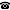# 2019 seminar talk: The Multiverse, Recursive Saturation and Well-Foundedness Mirage

Talk held by Michał Tomasz Godziszewski (Munich Center for Mathematical Philosophy, Universität München, Germany) at the KGRC seminar on 2019-10-17.

### Abstract

Recursive saturation, introduced by J. Barwise and J. Schlipf is a robust notion which has proved to be important for the study of nonstandard models (in particular, it is ubiquitous in the model theory of axiomatic theories of truth, e.g. in the topic of satisfaction classes, where one can show that if $M \models ZFC$ is a countable $\omega$-nonstandard model, then $M$ admits a satisfaction class iff $M$ is recursively saturated). V. Gitman and J. Hamkins showed in A Natural Model of the Multiverse Axioms that the collection of countable, recursively saturated models of set theory satisfy the so-called Hamkins's Multiverse Axioms. The property that forces all the models in the Multiverse to be recursively saturated is the so-called Well-Foundedness Mirage axiom which asserts that every universe is $\omega$-nonstandard from the perspective of some larger universe, or to be more precise, that: if a model $M$ is in the multiverse then there is a model $N$ in the multiverse such that $M$ is a set in $N$ and $N \models 'M$ is $\omega$-nonstandard$.'$. Inspection of the proof led to a question if the recursive saturation could be avoided in the Multiverse by weakening the Well-Foundedness Mirage axiom. Our main results answer this in the positive. We give two different versions of the Well-Foundedness Mirage axiom -- what we call Weak Well-Foundedness Mirage (saying that if $M$ is a model in the Multiverse then there is a model $N$ in the Multiverse such that $M \in N$ and $N \models 'M$ is nonstandard$.'$.) and Covering Well-Foundedness Mirage (saying that if $M$ is a model in the Multiverse then there is a model $N$ in the Multiverse with $K \in N$ such that $K$ is an end-extension of $M$ and $N \models 'K$ is $\omega$-nonstandard$.'$). I will present constructions of two different Multiverses satisfying these two weakened axioms.

This is joint work with V. Gitman. T. Meadows and K. Williams.

Here are the slides for this talk.

Kurt Gödel Research Center for Mathematical Logic. Währinger Straße 25, 1090 Wien, Austria.+43-1-4277-50501. Last updated: 2010-12-16, 04:37.